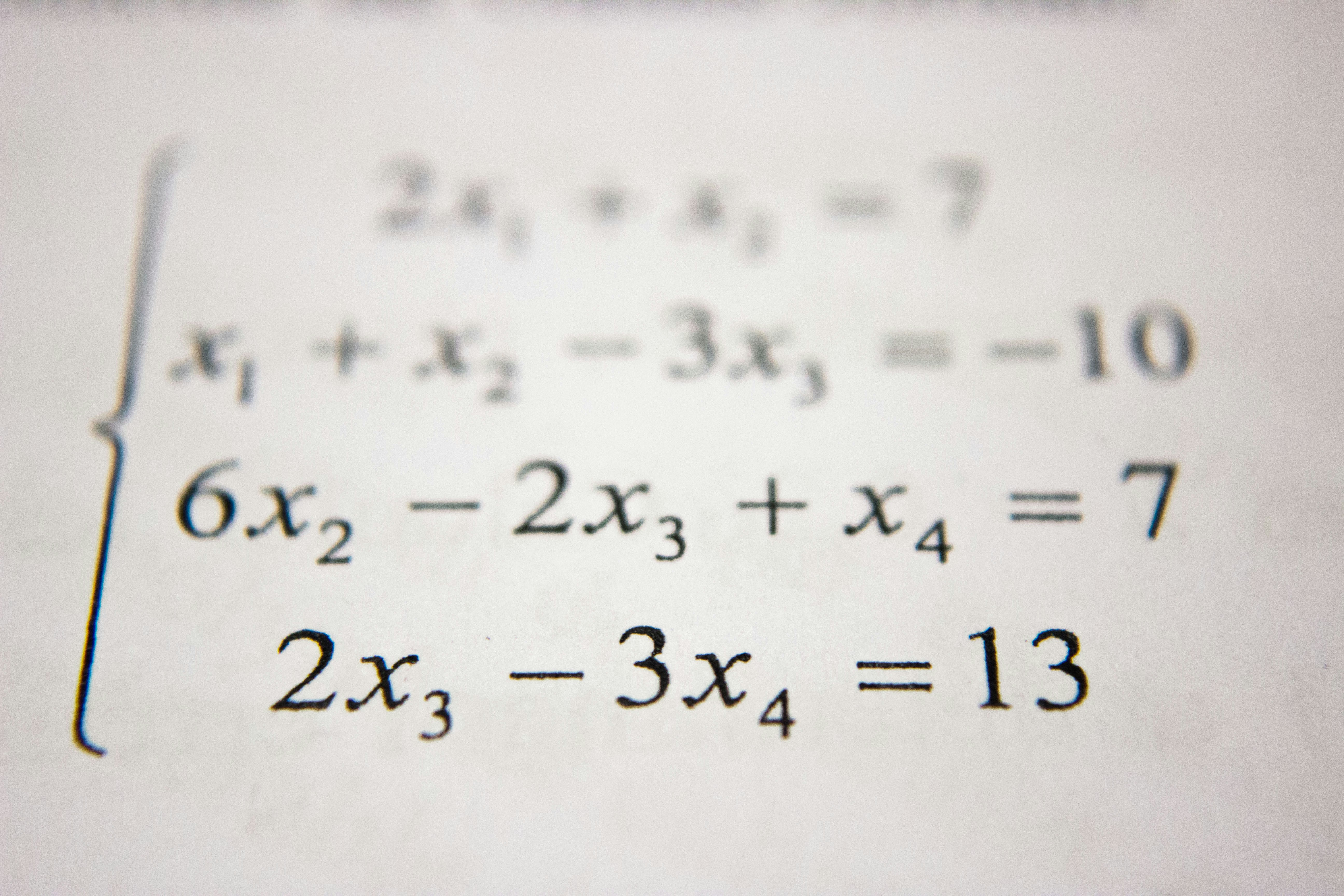Series

• Bijon Setyawan Raya

• February 13, 2022

10 minsRemember in the Linear Regression post, I mentioned that we can use a Gradient Descent algorithm to minimize the loss function, in this case the Mean Squared Error valuem, of a Linear Regression model. Let's see how it works at a glance.

Assume we have random data points like in the following graph.

We then make a regression line based on the following equation.

$y = \beta_0 + \beta_1 x$

At this point, we want to find the best value for $\beta_0$ and $\beta_1$. Assuming that we can't come up with the best answer, we can just randomly assign some random numbers to them. Now, let's set $\beta_0 = 0$ and $\beta_1 = -5$, and we are going to get a regression line in the following graph.

The problem is that we can't simply guess random numbers and plug them in to $\beta_0$ and $\beta_1$ over and over again. Clearly, we need a way to automate this.

Let's make a 2D graph showing the MSE graph where $\beta_0 = 0$ and $-10 \leq \beta_1 \leq 13$.

In the graph above, when $\beta_0 = 0$ and $\beta_1 = -5$, the MSE value is $217.42$ shown as a red dot.

I intentionally make the range wider to show everyone that the cost function (MSE) looks like an exponential function. It's not easy to tell where the minimum point is at this moment, but it could be around $-3 \leq \beta_1 \leq 3$. Therefore, we are not going to guess the suitable value $\beta_1$ one by one.

The ideal scenario is that we can have the red dot going down the valley slowly by itself.

However, we don't want the red dot to bounce here and there. If so, it means that the learning rate is too high. Don't worry if you are not sure what learning rate is since I have a seperate post explaning what it is.

In the next section, we are going to discuss how we can help our little friend The Little Red Dot going down the valley. Brace yourself because it is about to go down. I swear, no pun intended.

## Its Mathematics#

Since we want to minimize the MSE value of the Linear Regression model, we want to find the best value for $\beta_0$ and $\beta_0$ so that the regression line that is located as close to most data points as possible.

Let's express everything we want do in mathematical expressions.

The cost function

$J(\beta_0, \beta_1) = \frac{1}{N} \sum_{i=1}^N (f(x) - y_i)^2$

The objective function

$\min_{\beta_0, \beta_1} J(\beta_0, \beta_1)$

The update rules

$\Theta_{i} = \beta_i - \alpha \cdot \frac{\partial}{\partial \beta_i} J(\beta_0, \beta_1)$

where $\beta_i$ is the parameters we want to update and $\alpha$ is the learning rate.

Bear with me! This is going to involve a lot of Maths, especially Calculus.

Let's simplify the cost function that we want to minimize.

\begin{aligned} \frac{\partial}{\partial \beta_i} J(\beta_0, \beta_1) &= \frac{\partial}{\partial \beta_i} (\frac{1}{N} \sum_{i=1}^N (f(x) - y_i)^2) \\ &= \frac{1}{N} \frac{\partial}{\partial \beta_i} \sum_{i=1}^N (f(x) - y_i)^2 \end{aligned}

Solving the equality above with Power Rule, we then have

$\frac{\partial}{\partial \beta_i} J(\beta_0, \beta_1) = \frac{2}{N} \sum_{i=1}^N (f(x) - y_i) \frac{\partial}{\partial \beta_i} (f(x) - y_i)$

Since we want to update the $\beta_0$ and $\beta_1$ coefficients, we need to find the partial derivative of the cost function with respect to those coefficients.

\begin{aligned} \frac{\partial}{\partial \beta_0} J(\beta_0, \beta_1) &= \frac{2}{N} \sum_{i=1}^N (f(x) - y_i) \frac{\partial}{\partial \beta_0} (\beta_0 + \beta_1 x - y_i) \\ &= \frac{2}{N} \sum_{i=1}^N (f(x) - y_i) \end{aligned}
$\frac{\partial}{\partial \beta_1} J(\beta_0, \beta_1) = \frac{2}{N} \sum_{i=1}^N (f(x) - y_i) x$

We can remove the scalar $2$ from the two equations above by dividing the cost function, in this case the MSE equation, by $2$. Multiplying the cost function with a scalar will not affect how it's going to reach the minimum MSE value.

This new modified cost function is called One Half Mean Squared Error.

$J(\beta_0, \beta_1) = \frac{1}{2N} \sum_{i=1}^N (\hat{y}_i - y_i)^2$

Deriving the new cost function above, the scalar $2$ will be cancelled out from the partial derivations with respect to $\beta_0$ and $\beta_1$,

\begin{aligned} \frac{\partial}{\partial \beta_0} J(\beta_0, \beta_1) = \frac{1}{N} \sum_{i=1}^N (f(x) - y_i) \\ \frac{\partial}{\partial \beta_1} J(\beta_0, \beta_1) = \frac{1}{N} \sum_{i=1}^N (f(x) - y_i) x \end{aligned}

Plugging each of the equation above into the update rules with respect to those coefficients, we get

\begin{aligned} \beta_0 = \beta_0 - \alpha \cdot \frac{1}{N} \sum_{i=1}^N (f(x) - y_i) \\ \beta_1 = \beta_1 - \alpha \cdot \frac{1}{N} \sum_{i=1}^N (f(x) - y_i) x \end{aligned}

The two equations above will help us to approximate the minimum value of the cost function by updating $\beta_0$ and $\beta_1$ over time.

## Conclusion#

Here are the takeaway keypoints from this entire post.

1. Gradient Descent is a technique that helps a model get better at future predictions.
2. The cost function
$J(\beta_0, \beta_1) = \frac{1}{2N} \sum_{i=1}^N (\hat{y}_i - y_i)^2$
3. The update rule for $\beta_0$
$\beta_0 = \beta_0 - \alpha \cdot \frac{1}{N} \sum_{i=1}^N (f(x) - y_i)$
4. The update rule for $\beta_1$
$\beta_1 = \beta_1 - \alpha \cdot \frac{1}{N} \sum_{i=1}^N (f(x) - y_i)x$
5. The weights $\beta_0$ and $\beta_1$ are updated after all the data points are processed.
6. The learning rate $\alpha$ is a hyperparameter that controls the speed of the gradient descent on any slopes.
7. High learning rate may cause the model's MSE to bounce here and there or to converge at a suboptimial minimum.
8. Low learning rate will cause the model to stuck in a suboptimial minimum.

In the next part, we are going to implement Batch Gradient Descent using the mathematical equations that we have derived. See you in the next one.